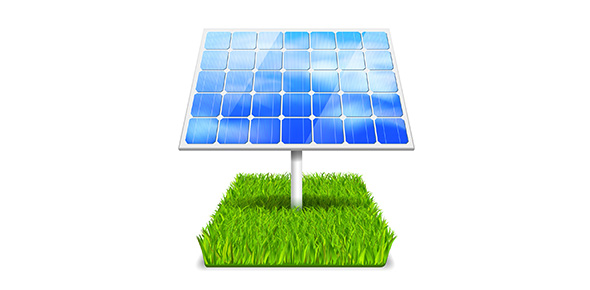# Mr Fays Energy Quiz

27 Questions | Total Attempts: 111SettingsTesting your understanding of Chapter 35 of Science Matters.

• 1.
.Which of the following is the best example of increasing an object's potential energy?
• A.

rolling a bowling ball

• B.

turning on a light bulb

• C.

stretching a rubber band

• D.

dropping a pencil

• 2.
An object that has kinetic energy must be...
• A.

lifted above earth's surface.

• B.

in motion.

• C.

at rest.

• D.

None of the above.

• 3.
. Sound energy is...
• A.

the energy of a compound that changes as its atoms are rearranged to form new compounds

• B.

the total energy of the particles that make up an object.

• C.

The energy caused by an object's vibrations.

• D.

the energy of motion.

• 4.
Which of the following convert chemical energy to mechanical energy?
• A.

human

• B.

Car

• C.

Jet Ski

• D.

All of above

• 5.
Which of the following is a conversion from chemical energy to thermal energy?
• A.

Coal is burned to boil water.

• B.

Food is digested and used to regulate body temperature.

• C.

Charcoal is burned in a barbeque pit.

• D.

All of above

• 6.
As height increases, so does...
• A.

thermal energy.

• B.

Mechanical energy.

• C.

kinetic energy.

• D.

potential energy.

• 7.
The law of _________________ of energy states that energy cannot be created or destroyed.
• A.

transformation

• B.

absorption

• C.

conservation

• D.

stability

• 8.
According to the law of conservation of energy, in theory, a bouncy ball should never stop bouncing. However, we know that it eventually stops. Where does the energy go.
• A.

some gets converted into sound energy and escapes into the surroundings.

• B.

some gets converted into thermal energy and escapes into the surroundings.

• C.

Both A and B

• 9.
Which one is not a fossil fuel
• A.

Oil

• B.

Wood

• C.

Coal

• 10.
What percentage of the Energy used around the world comes from Fossil Fuels
• A.

None

• B.

90%

• C.

40%

• 11.
Today, which renewable energy source provides Ireland. with the most energy?
• A.

Wind

• B.

Solar

• C.

Geothermal

• D.

Hydro

• 12.
Electricity is the movement of
• A.

Atoms

• B.

Molecules

• C.

Electrons

• D.

Neutrons

• 13.
Most of the energy we use originially came from the
• A.

Sun

• B.

Air

• C.

Soil

• D.

Oceans

• 14.
Electrical Energy can be produced from
• A.

Mechanical energy

• B.

Chemical energy

• C.

• D.

All of the above

• 15.
In a nuclear power plant, uranium atoms
• A.

Combine and give off heat energy

• B.

Split and give off heat energy

• C.

Burn and give off heat energy

• D.

Split and give offelectrons

• 16.
Which of these energy changes take take place in a flashlight
• A.

Electrical to chemical to light to heat

• B.

Electrical to light to heat

• C.

Heat to light

• D.

Chemical to electrical to light to heat.

• 17.
One disadvantage of Nuclear Fission is
• A.

Its really smelly

• B.

• C.

The temperature required to start the reaction

• D.

The lack of deuterium on earth.

• 18.
Which answer means energy on the move.
• A.

Kinetic Energy

• B.

Potential Energy

• C.

Nuclear Energy

• D.

Chemical Energy

• 19.
Which of these is a renewable energy source
• A.

Oil

• B.

Wind

• C.

Nuclear Energy

• D.

Gas

• 20.
The Unit of Energy is
• A.

Watt

• B.

Joule

• C.

Volt

• D.

Ampere

• 21.
The best definition of enegry is
• A.

Something you can run out of

• B.

The ability to do work

• C.

The power of a force

• D.

Something you need to live

• 22.
In what forms is some energy usually lost when being transformed from one form to another
• A.

Heat and sound

• B.

Heat and light

• C.

Sound and light

• D.

Heat and water

• 23.
Which is the main energy transfer happening inside a car engine (just the engine part)
• A.

Chemical to heat

• B.

Kinetic to heat

• C.

Heat to kinetic

• D.

Chemical to sound

• 24.
Energy is the same thing as Power
• A.

True

• B.

False

• 25.
If you increase the velocity of and object  the kinetic energy will
• A.

Increase

• B.

Decrease

• C.

Stay the same

Related TopicsBack to top